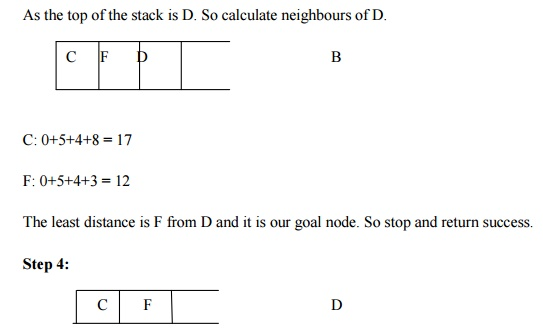Home | | Artificial Intelligence | Branch and Bound Search: Concept, Algorithm, Implementation, Advantages, Disadvantages

## Chapter: Artificial Intelligence

Branch and Bound is an algorithmic technique which finds the optimal solution by keeping the best solution found so far. If partial solution canâ€™t improve on the best it is abandoned, by this method the number of nodes which are explored can also be reduced.

Branch and Bound Search

Branch and Bound is an algorithmic technique which finds the optimal solution by keeping the best solution found so far. If partial solution canâ€™t improve on the best it is abandoned, by this method the number of nodes which are explored can also be reduced. It also deals with the optimization problems over a search that can be presented as the leaves of the search tree. The usual technique for eliminating the sub trees from the search tree is called pruning. For Branch and Bound algorithm we will use stack data structure.

Concept:

Step 1: Traverse the root node.

Step 2: Traverse any neighbour of the root node that is maintaining least distance from the root node.

Step 3: Traverse any neighbour of the neighbour of the root node that is maintaining least distance from the root node.

Step 4: This process will continue until we are getting the goal node.

Algorithm:

Step 1: PUSH the root node into the stack.

Step 2: If stack is empty, then stop and return failure.

Step 3: If the top node of the stack is a goal node, then stop and return success.

Step 4: Else POP the node from the stack. Process it and find all its successors. Find out the path containing all its successors as well as predecessors and then PUSH the successors which are belonging to the minimum or shortest path.

Step 5: Go to step 5.

Step 6: Exit.

Implementation:

Let us take the following example for implementing the Branch and Bound algorithm.Hence the searching path will be A-B -D-F

As it finds the minimum path instead of finding the minimum successor so there should not be any repetition.

The time complexity is less compared to other algorithms.

The load balancing aspects for Branch and Bound algorithm make it parallelization difficult.

The Branch and Bound algorithm is limited to small size network. In the problem of large networks, where the solution search space grows exponentially with the scale of the network, the approach becomes relatively prohibitive.

Study Material, Lecturing Notes, Assignment, Reference, Wiki description explanation, brief detail
Artificial Intelligence : Branch and Bound Search: Concept, Algorithm, Implementation, Advantages, Disadvantages |